Documentation

This is machine translation

Mouseover text to see original. Click the button below to return to the English version of the page.

Signal Analyzer

Visualize and compare multiple signals and spectra

Description

The Signal Analyzer app is an interactive tool for visualizing, preprocessing, measuring, analyzing, and comparing signals in the time domain, in the frequency domain, and in the time-frequency domain. Using the app, you can:

• Easily access all the signals in the MATLAB® workspace

• Smooth, filter, resample, detrend, duplicate, extract, and rename signals without leaving the app

• Add and apply custom preprocessing functions

• Visualize and compare multiple waveform, spectrum, persistence, spectrogram, and scalogram representations of signals simultaneously

• Label signals interactively for analysis or for machine learning and deep learning applications

The Signal Analyzer app provides a way to work with many signals of varying durations at the same time and in the same view.

You need a Wavelet Toolbox™ license to use the scalogram view.Open the Signal Analyzer App

• MATLAB Toolstrip: On the Apps tab, under Signal Processing and Communications, click the app icon.

• MATLAB command prompt: Enter `signalAnalyzer`.

Examples

expand all

Implement a basic digital music synthesizer and use it to play a traditional song in a three-voice arrangement. Specify a sample rate of 2 kHz. Save the song as a MATLAB® timetable.

```fs = 2e3; t = 0:1/fs:0.3-1/fs; l = [0 130.81 146.83 164.81 174.61 196.00 220 246.94]; m = [0 261.63 293.66 329.63 349.23 392.00 440 493.88]; h = [0 523.25 587.33 659.25 698.46 783.99 880 987.77]; note = @(f,g) [1 1 1]*sin(2*pi*[l(g) m(g) h(f)]'.*t); mel = [3 2 1 2 3 3 3 0 2 2 2 0 3 5 5 0 3 2 1 2 3 3 3 3 2 2 3 2 1]+1; acc = [3 0 5 0 3 0 3 3 2 0 2 2 3 0 5 5 3 0 5 0 3 3 3 0 2 2 3 0 1]+1; song = []; for kj = 1:length(mel) song = [song note(mel(kj),acc(kj)) zeros(1,0.01*fs)]; end song = song'/(max(abs(song))+0.1); % To hear, type sound(song,fs) tune = timetable(seconds((0:length(song)-1)'/fs),song);```

Open Signal Analyzer and drag the timetable from the Workspace browser to the Signal table. Click Display Grid ▼ to create a two-by-two grid of displays. Select the top two displays and the lower left display and click the Spectrum button to add a spectrum view. Select the lower right display, click Time-Frequency to add a spectrogram view, and click Time to remove the time view. Drag the song to all four displays. Select the lower right display, and in the Spectrogram tab, specify a time resolution of 0.31 second (310 ms) and 0% overlap between adjoining segments. Set the Power Limits to $-50$ dB and $-10$ dB.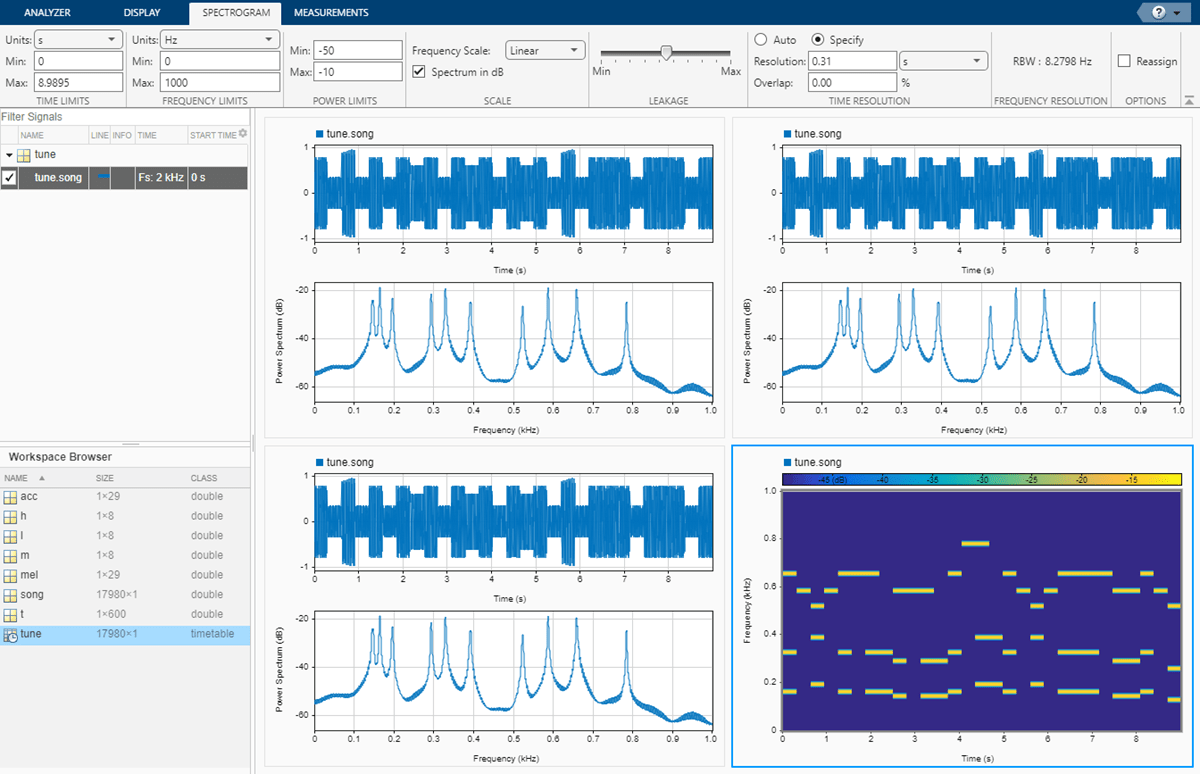On the Analyzer tab, click Duplicate three times to create three copies of the song. Rename the copies as `high`, `medium`, and `low` by double-clicking the Name column in the Signal table. Move the copies to the top two and lower left displays.

Preprocess the duplicate signals using filters.

1. Select the `high` signal by clicking its name in the Signal table. On the Analyzer tab, click Highpass. On the Highpass tab that appears, enter a passband frequency of 450 Hz and increase the steepness to 0.95. Click Highpass.

2. Select the `medium` signal by clicking its name in the Signal table. On the Analyzer tab, click Preprocessing ▼ and select Bandpass. On the Bandpass tab that appears, enter 230 Hz and 450 Hz as the lower and upper passband frequencies, respectively. Increase the steepness to 0.95. Click Bandpass.

3. Select the `low` signal by clicking its name in the Signal table. On the Analyzer tab, click Lowpass. On the Lowpass tab that appears, enter a passband frequency of 230 Hz and increase the steepness to 0.95. Click Lowpass.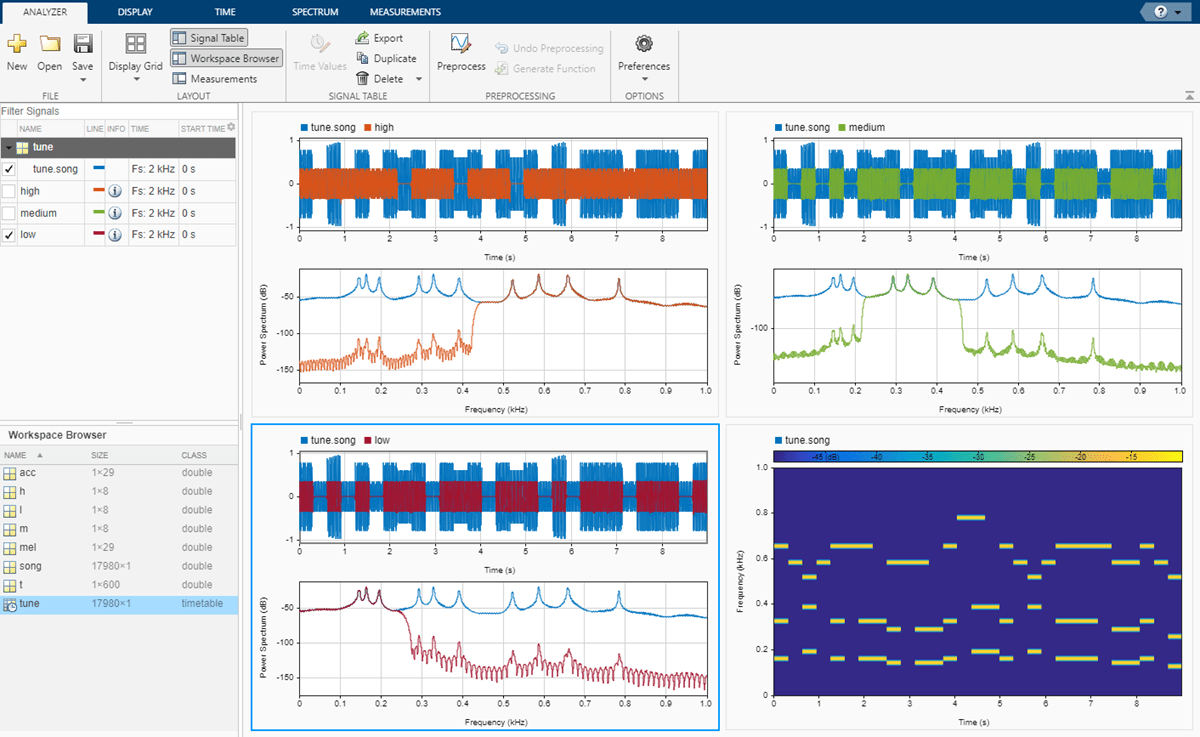On each of the three displays containing filtered signals:

1. Remove the original signal by clearing the check box next to its name.

2. On the Display tab, click Time-Frequency to add a spectrogram view and click Time to remove the time view.

3. On the Spectrogram tab, specify a time resolution of 0.31 second and 0% overlap between adjoining segments. Set the Power Limits to $-50$ dB and $-10$ dB.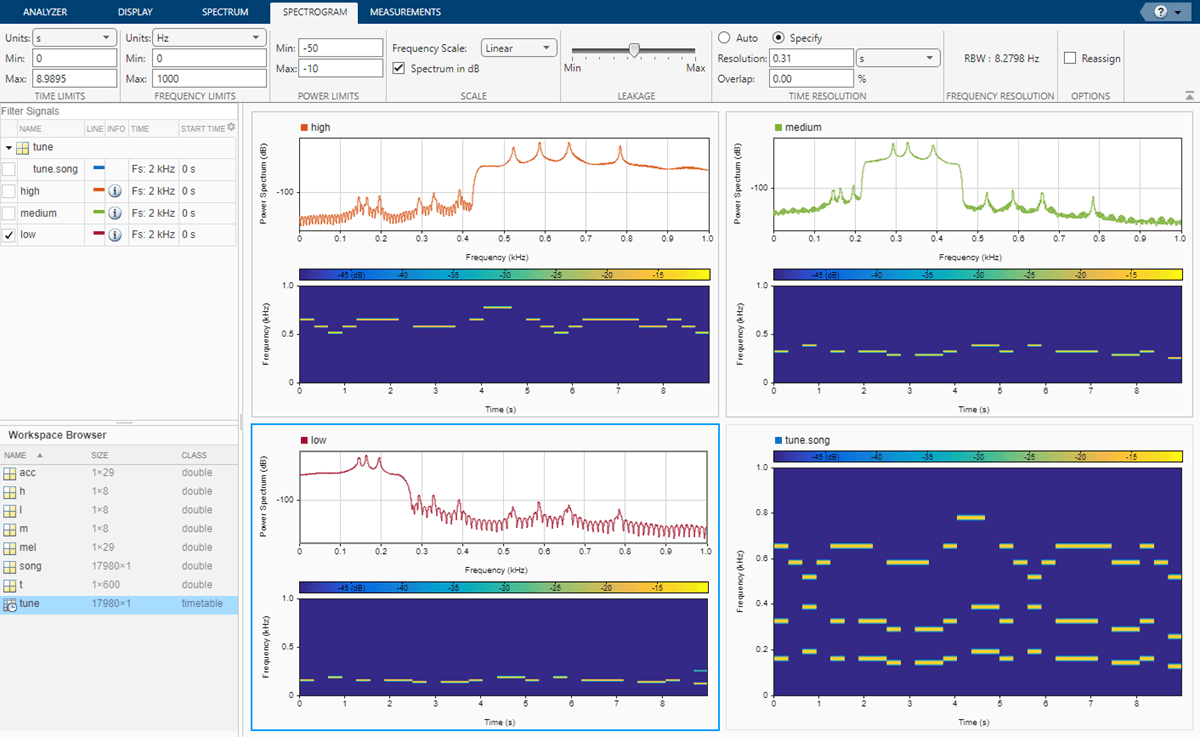Select the three filtered signals by clicking their Name column in the Signal table. On the Analyzer tab, click Export and save the signals to a MAT-file called `music.mat`. In MATLAB, load the file to the workspace. Plot the spectra of the three signals.

```load music pspectrum(low) hold on pspectrum(medium) pspectrum(high) hold off```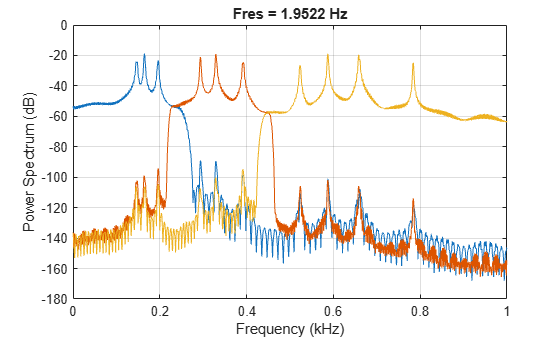```% To hear the different voices, type % sound(low.Var1,fs), pause(5), sound(medium.Var1,fs), pause(5), sound(high.Var1,fs)```

Load a file that contains audio data from a Pacific blue whale, sampled at 4 kHz. The file is from the library of animal vocalizations maintained by the Cornell University Bioacoustics Research Program. The time scale in the data is compressed by a factor of 10 to raise the pitch and make the calls more audible. Convert the signal to a MATLAB® timetable.

```whaleFile = fullfile(matlabroot,'examples','matlab','bluewhale.au'); [w,fs] = audioread(whaleFile); whale = timetable(seconds((0:length(w)-1)'/fs),w); % To hear, type soundsc(w,fs)```

Open Signal Analyzer and drag the timetable to a display. Four features stand out from the noise. The first is known as a trill, and the other three are known as moans.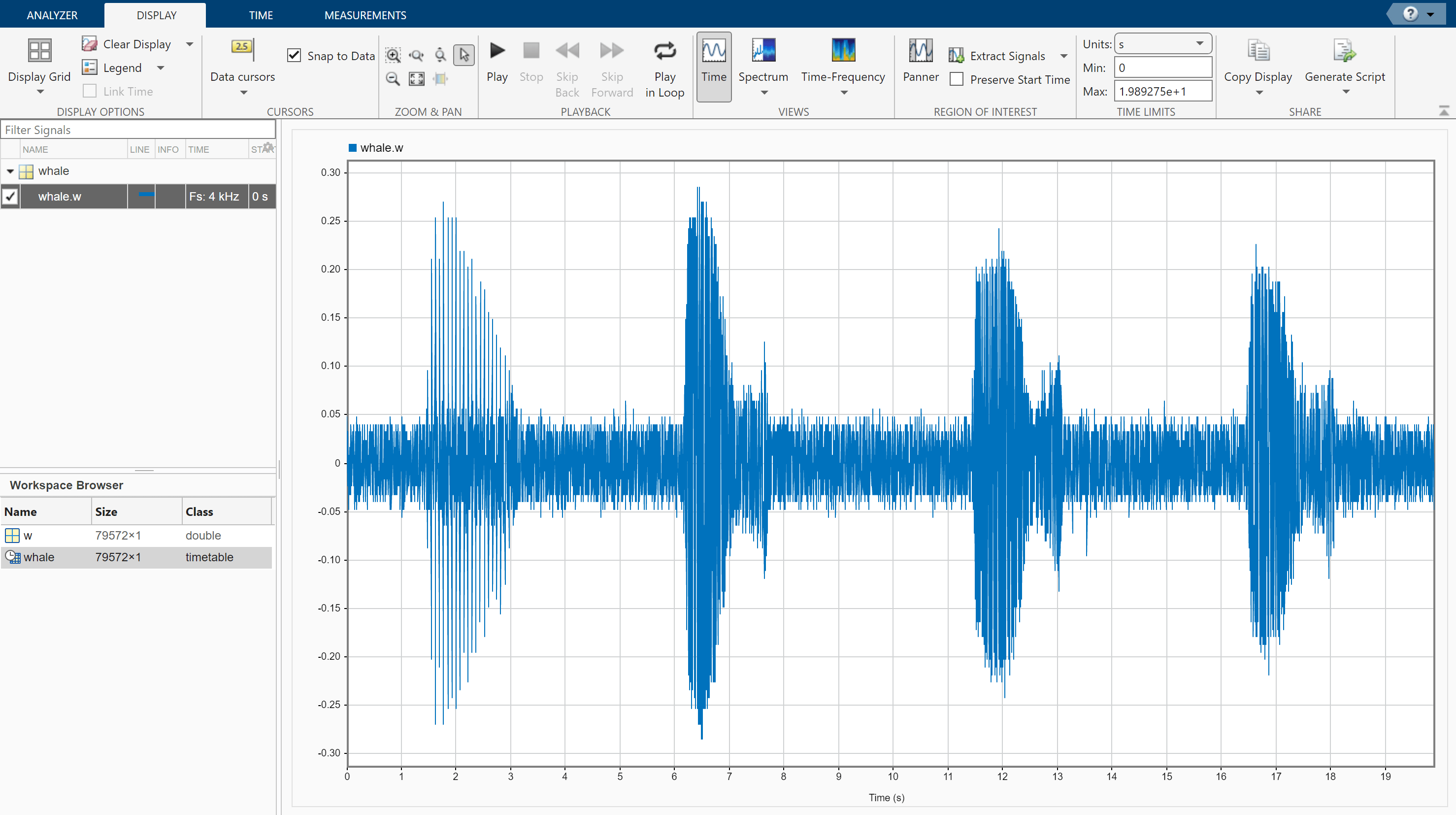On the Display tab, click Spectrum to open a spectrum view and click Panner to activate the panner. Use the panner to create a zoom window with a width of about 2 seconds. Drag the zoom window so that it is centered on the trill. The spectrum shows a noticeable peak at around 900 Hz.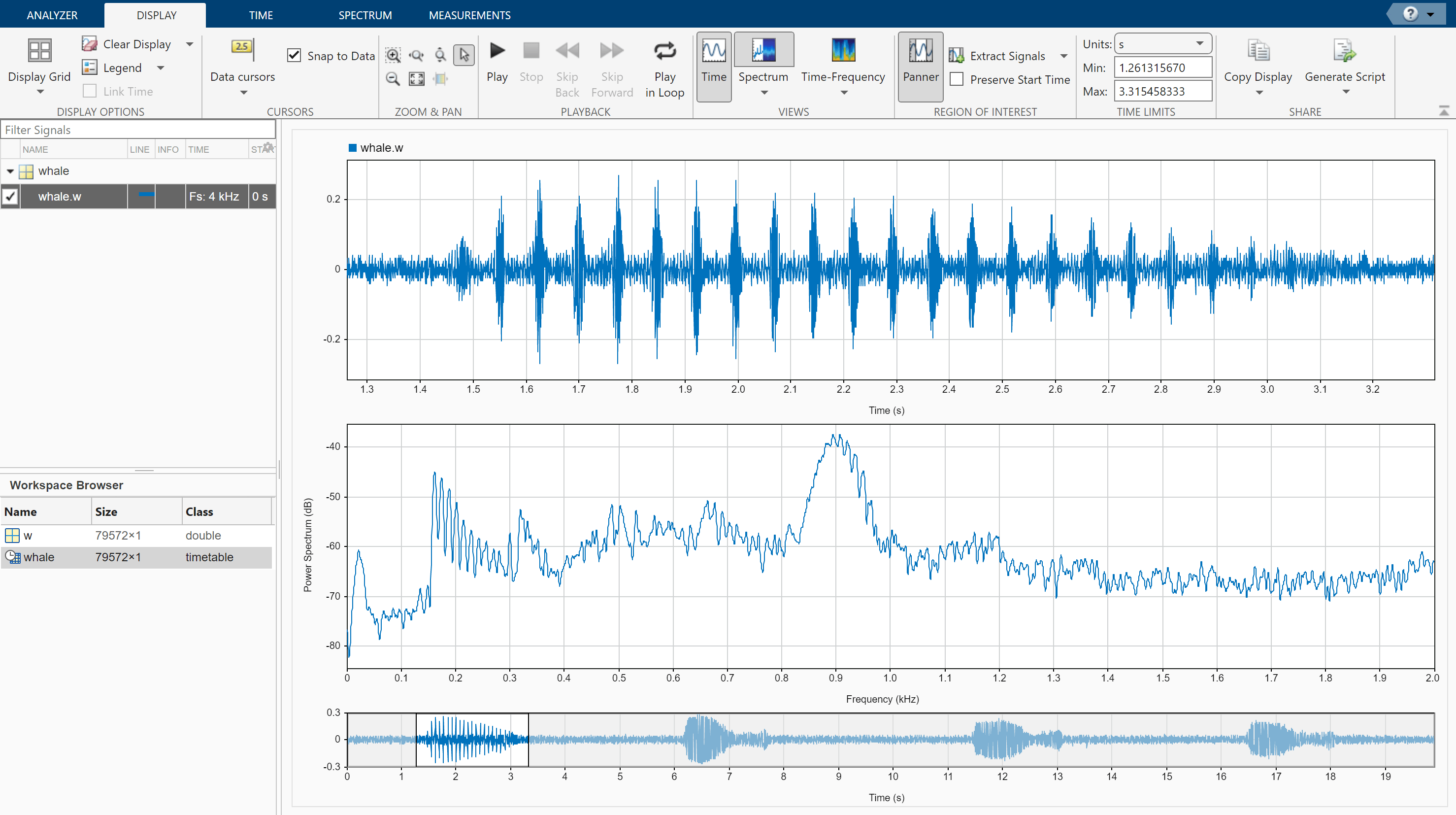Extract the three moans to compare their spectra:

1. Center the panner zoom window on the first moan. The spectrum has eight clearly defined peaks, located very close to multiples of 170 Hz. Click Extract Signals ▼ and select `Between Time Limits`.

2. Click Panner to hide the panner. Press the space bar to see the full signal. Click Zoom in X and zoom in on a 2-second interval of the time view centered on the second moan. The spectrum again has peaks at multiples of 170 Hz. Click Extract Signals ▼ and select `Between Time Limits`.

3. Press the space bar to see the full signal. Click Data Cursors ▼ and select `Two`. Place the time-domain cursors in a 2-second interval around the third moan. Again, there are peaks at multiples of 170 Hz. Click Extract Signals ▼ and select `Between Time Cursors`.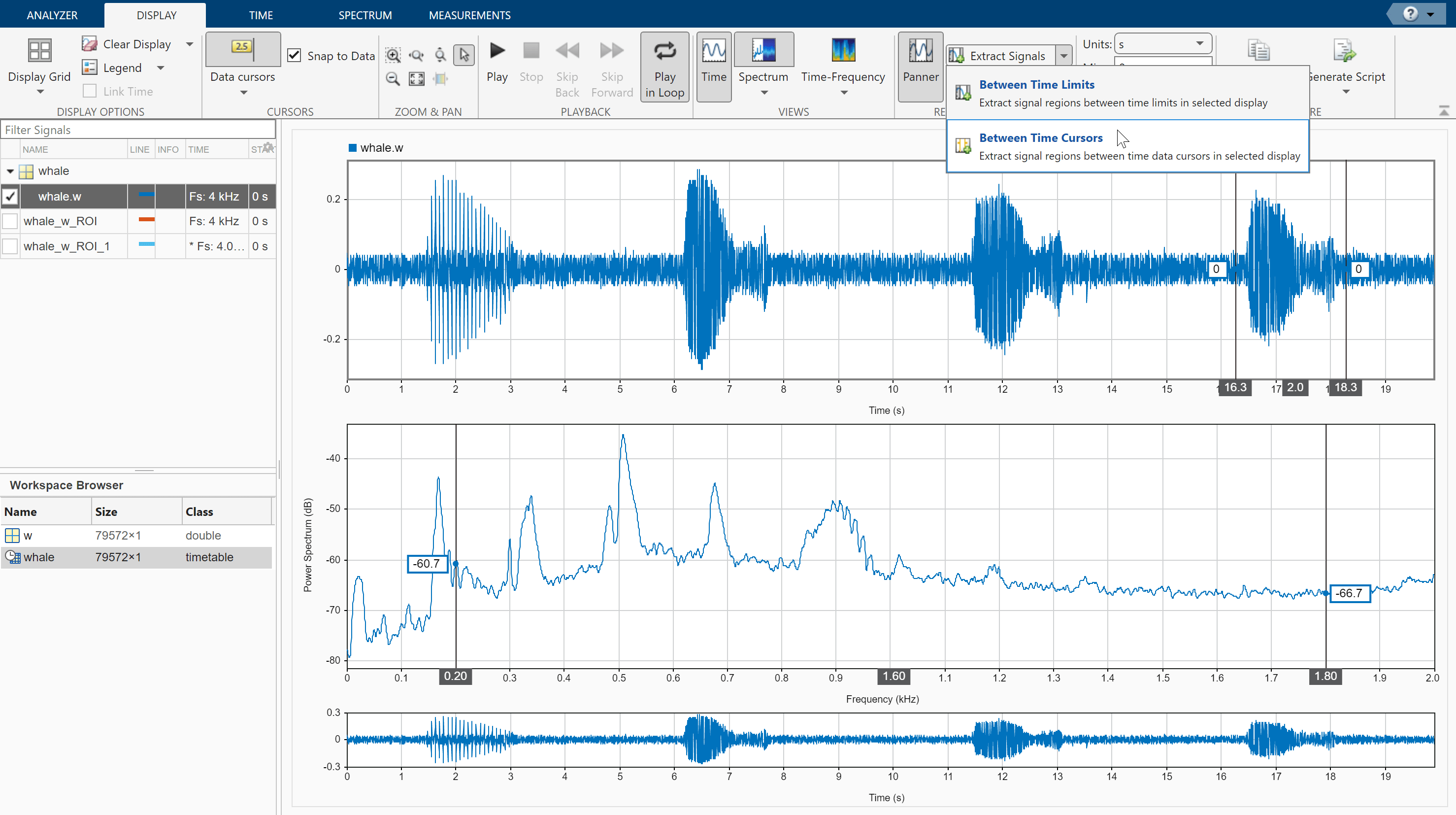Remove the original signal from the display by clearing the check box next to its name in the Signal table. Display the three regions of interest you just extracted. Their spectra lie approximately on top of each other. Move the frequency-domain cursors to the locations of the first and third spectral peaks. Asterisks in cursor labels indicate interpolated signal values.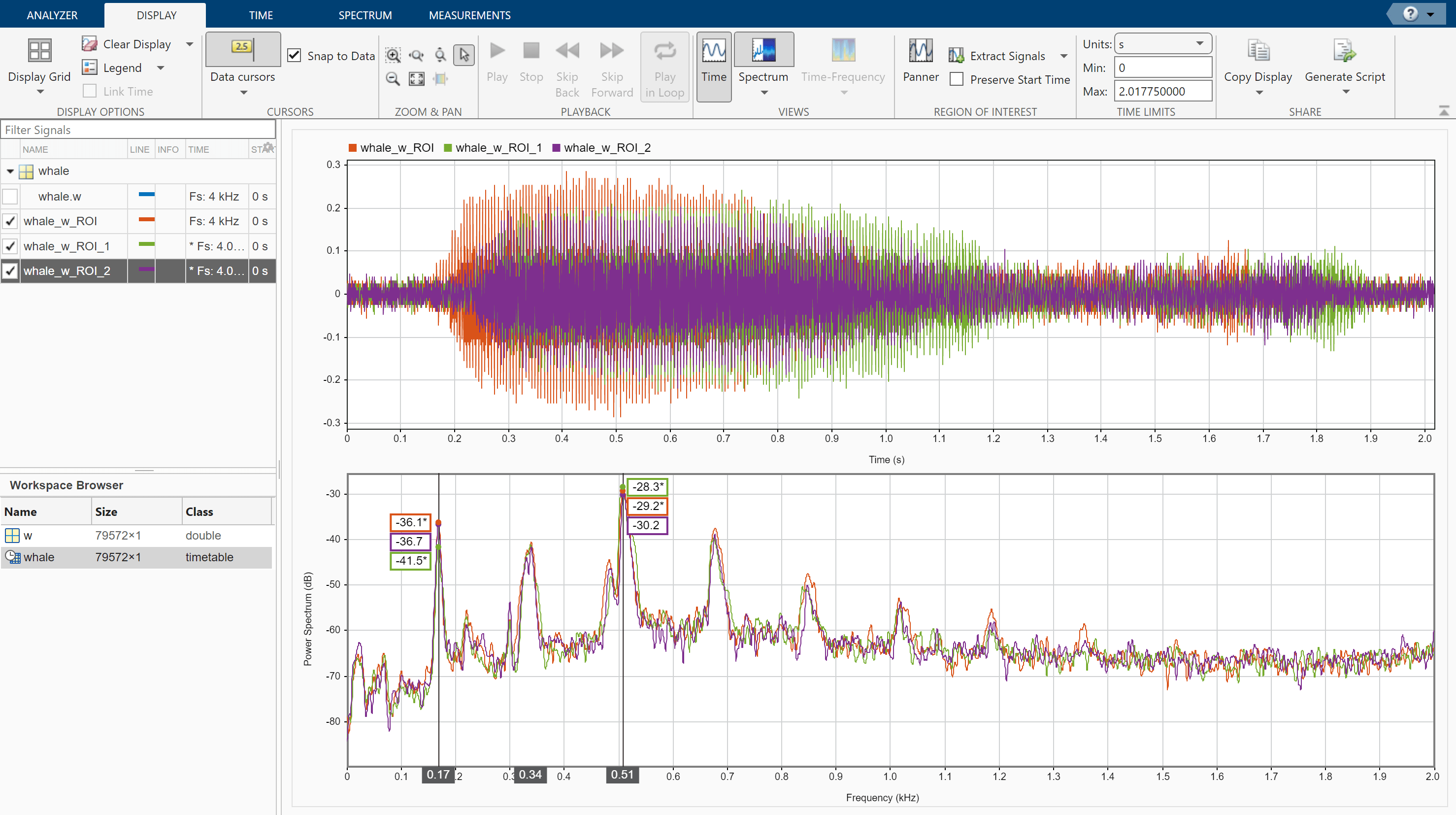Load a datafile containing an echolocation pulse emitted by a big brown bat (Eptesicus fuscus) and measured with a sampling interval of 7 microseconds. Create a MATLAB® timetable using the signal and the time information.

```load batsignal t = (0:length(batsignal)-1)*DT; sg = timetable(seconds(t)',batsignal);```

Open Signal Analyzer and drag the timetable from the Workspace browser to the Signal table. Click Display Grid ▼ to create two side-by-side displays. Select each display and click the Time-Frequency button to add a spectrogram view.

Drag the timetable to both displays.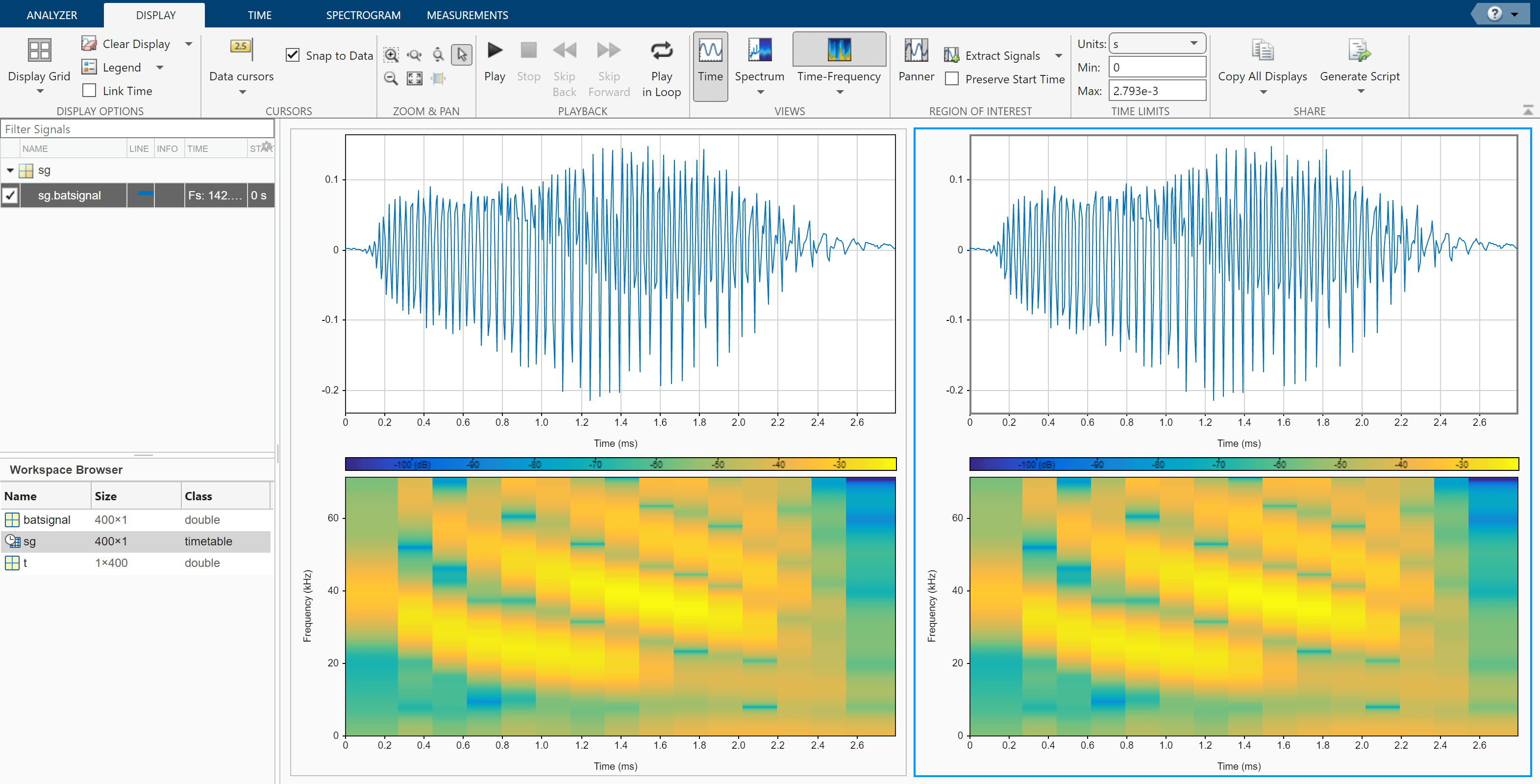Select the Spectrogram tab. On the display at right, check Reassign. For each display:

• Set the time resolution to 280 microseconds and specify 85% overlap between adjoining segments.

• Use the Leakage slider to increase the leakage until the RBW is about 4.5 kHz.

• Set the power limits to –45 dB and –20 dB.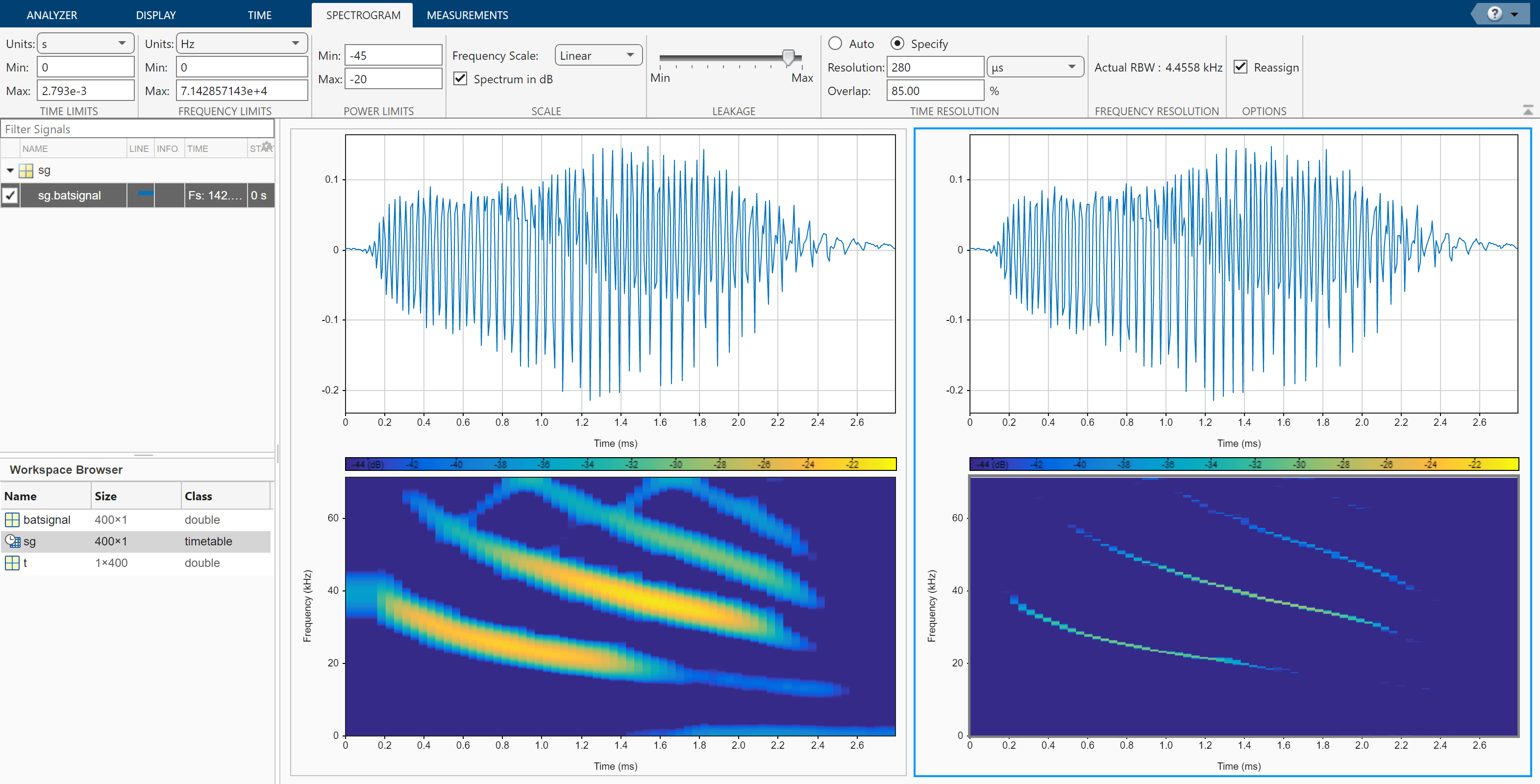The reassigned spectrogram clearly shows three time-frequency ridges. To track the ridges, select the display at right. On the Display tab, click Generate Script and select `Spectrogram Script`. The script appears in the Editor.

```% Compute spectrogram % Generated by MATLAB(R) 9.7 and Signal Processing Toolbox 8.2. % Generated on: 26-Dec-2018 17:21:44 % Parameters timeLimits = seconds([0 0.002793]); % seconds frequencyLimits = [0 71428.57]; % Hz leakage = 0.9; timeResolution = 0.00028; % seconds overlapPercent = 85; reassignFlag = true; %% % Index into signal time region of interest sg_batsignal_ROI = sg(:,'batsignal'); sg_batsignal_ROI = sg_batsignal_ROI(timerange(timeLimits(1),timeLimits(2),'closed'),1); % Compute spectral estimate % Run the function call below without output arguments to plot the results [P,F,T] = pspectrum(sg_batsignal_ROI, ... 'spectrogram', ... 'FrequencyLimits',frequencyLimits, ... 'Leakage',leakage, ... 'TimeResolution',timeResolution, ... 'OverlapPercent',overlapPercent, ... 'Reassign',reassignFlag);```

Run the script. Plot the reassigned spectrogram.

```mesh(seconds(T),F,P) xlabel('Time') ylabel('Frequency') axis tight view(2) colormap pink```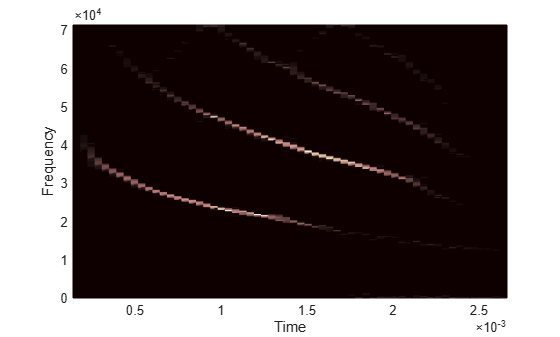Use the `tfridge` function to track the ridges.

```[fridge,~,lridge] = tfridge(P,F,0.01,'NumRidges',3,'NumFrequencyBins',10); hold on plot3(seconds(T),fridge,P(lridge),':','linewidth',3) hold off```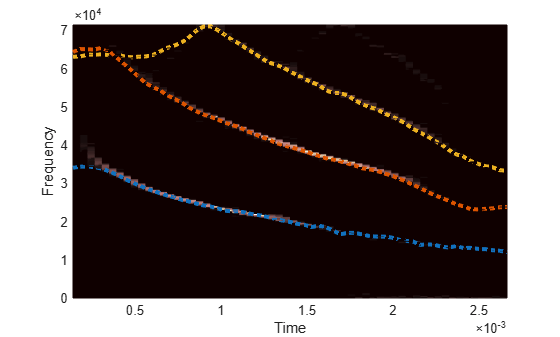Thanks to Curtis Condon, Ken White, and Al Feng of the Beckman Center at the University of Illinois for the bat data and permission to use it in this example.

Sensors can return clipped readings if the data are larger than a given saturation point. To reconstruct the readings, you can fit a polynomial through the points adjacent to the saturated intervals. Write a function that performs the reconstruction and integrate it into Signal Analyzer.

Generate a three-channel signal sampled at 1 kHz for 14 seconds. The signal has several peaks of varying sizes and shapes. A sensor that reads the signal saturates at 0.1 V.

```fs = 1000; t = 0:1/fs:14-1/fs; sig = [chirp(t-1,0.1,17,2,'quadratic',1).*sin(2*pi*t/5); chirp(t-2,2,2,2.1,'quadratic',100).*exp(-(t-6.5).^2/20).*sin(2*pi*t*2); 0.85*besselj(0,5*(sin(2*pi*(t+1.5).^2/20).^2)).*sin(2*pi*t/9)]'; sigsat = sig; stv = 0.1; sigsat(sigsat >= stv) = stv;```

Open Signal Analyzer and drag the original signal and the saturated signal to the Signal table. Drag each original and saturated channel to its own display.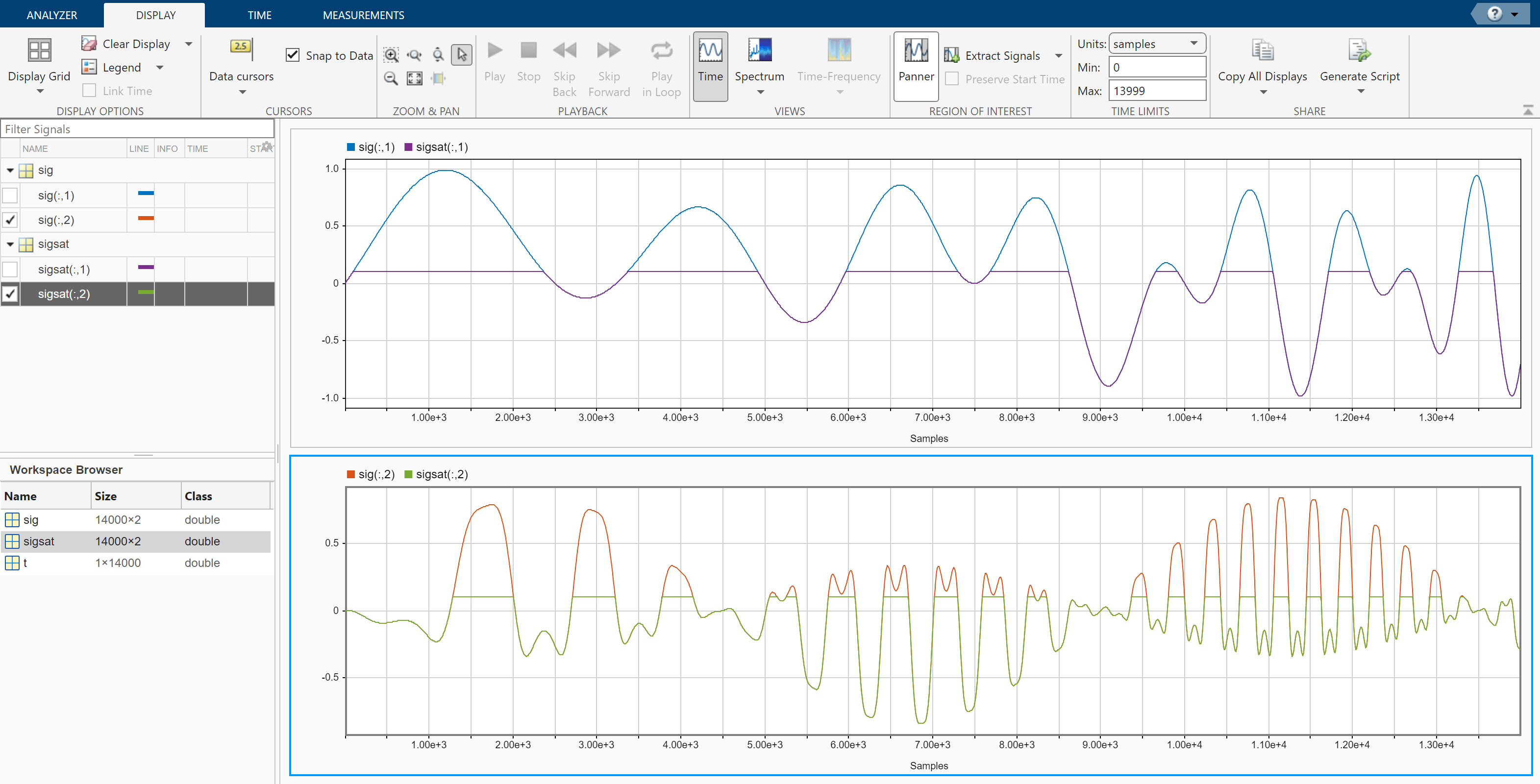Write a function that uses a polynomial to reconstruct the signal peaks:

• The first input argument, `x`, is the input signal. This argument must be a vector and is treated as a single channel.

• The second input argument, `tIn`, is a vector of time values. The vector must have the same length as the signal. If the input signal has no time information, the function reads this argument as an empty array.

• Use `varargin` to specify additional input arguments. If you do not have additional input arguments, you can omit `varargin`. Enter the additional arguments as an ordered comma-separated list in the Preprocess tab.

• The first output argument, `y`, is the preprocessed signal.

• The second output argument, `tOut`, is a vector of output time values. If the input signal has no time information, `tOut` is returned as an empty array.

• To implement your algorithm, you can use any MATLAB® or Signal Processing Toolbox™ function.

```function [y,tOut] = declip(x,tIn,varargin) % Declip saturated signal by fitting a polynomial % Initialize the output signal y = x; % For signals with no time information, use sample numbers as abscissas if isempty(tIn) tOut = []; t = (1:length(x))'; else t = tIn; tOut = t; end % Specify the degree of the polynomial as an optional input argument % and provide a default value of 4 if nargin<3 ndx = 4; else ndx = varargin{1}; end % To implement your algorithm, you can use any MATLAB or Signal % Processing Toolbox function % Find the intervals where the signal is saturated and generate an % array containing the interval endpoints idx = find(x==max(x)); fir = [true;diff(idx)~=1]; ide = [idx(fir) idx(fir([2:end 1]))]; % For each interval, fit a polynomial of degree ndx over the ndx+1 points % before the interval and the ndx+1 points after the interval for k = 1:size(ide,1) bef = ide(k,1); aft = ide(k,2); intv = [bef-1+(-ndx:0) aft+1+(0:ndx)]; [pp,~,mu] = polyfit(t(intv),x(intv),ndx); y(bef:aft) = polyval(pp,t(bef:aft),[],mu); end end```

Add the function to Signal Analyzer as a custom preprocessing function. On the Analyzer tab, click Preprocessing ▼ and select Add Custom Function. Input the function name and description. Paste the text of your function in the editor window that appears. Save the file. The function appears in the preprocessing gallery.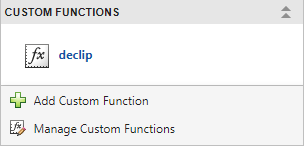Demonstrate that the function you created reconstructs the saturated regions.

1. Select the first channel of the saturated signal in the Signal table.

2. On the Analyzer tab, click Preprocessing ▼ and select declip.

3. On the Preprocessing tab that appears, click Preprocess.

Verify that the preprocessing function works when the signals have time information.

1. Select `sig` and `sigsat` in the Signal table. Do not select individual channels.

2. On the Analyzer tab, click Time Values, select `Sample Rate and Start Time`, and specify `fs` as the sample rate.

3. The signal in the top panel, including the reconstructed regions, has time information.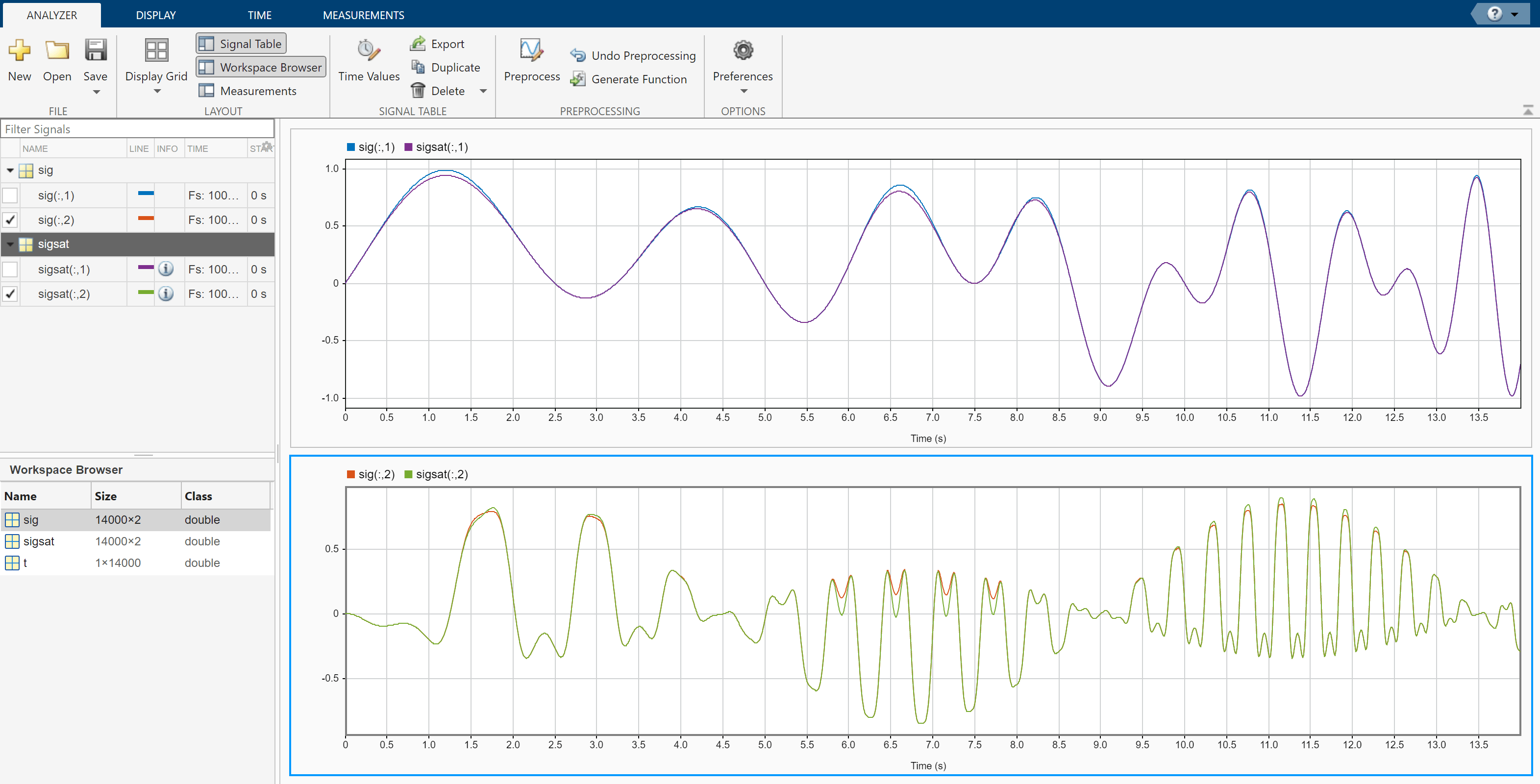Check that the function works when you specify optional inputs.

1. Select the second and third channels of the saturated signal in the Signal table.

2. On the Preprocessing tab, enter `8` in the Arguments field and click Preprocess. The preprocessing function uses a polynomial of degree 8 to reconstruct the saturated regions.Use Signal Analyzer to compute the envelope spectrum of a bearing vibration signal and look for defects. Generate MATLAB® scripts and functions to automate the analysis.

Generate Bearing Vibration Data

A bearing with the dimensions shown in the figure is driven at ${\mathit{f}}_{0}=25$ cycles per second. An accelerometer samples the bearing vibrations at 10 kHz.Generate vibration signals from two defective bearings using the `bearingdata` function at the end of the example. In one of the signals, `xBPFO`, the bearing has a defect in the outer race. In the other signal, `xBPFI`, the bearing has a defect in the inner race. For more details on modeling and diagnosing defects in bearings, see Vibration Analysis of Rotating Machinery and `envspectrum`.

`[t,xBPFO,xBPFI,bpfi] = bearingdata;`

Compute Envelope Spectrum Using Signal Analyzer

Open Signal Analyzer and drag the BPFO signal to a display. Add time information to the signal by selecting it in the Signal table and clicking the Time Values button on the Analyzer tab. Select the `Sample Rate and Start Time` option and enter the 10 kHz sample rate.

On the Display tab, click Spectrum to open a spectrum view. The spectrum of the vibration signal shows BPFO harmonics modulated by the 3 kHz impact frequency. At the low end of the spectrum, the driving frequency and its orders obscure other features.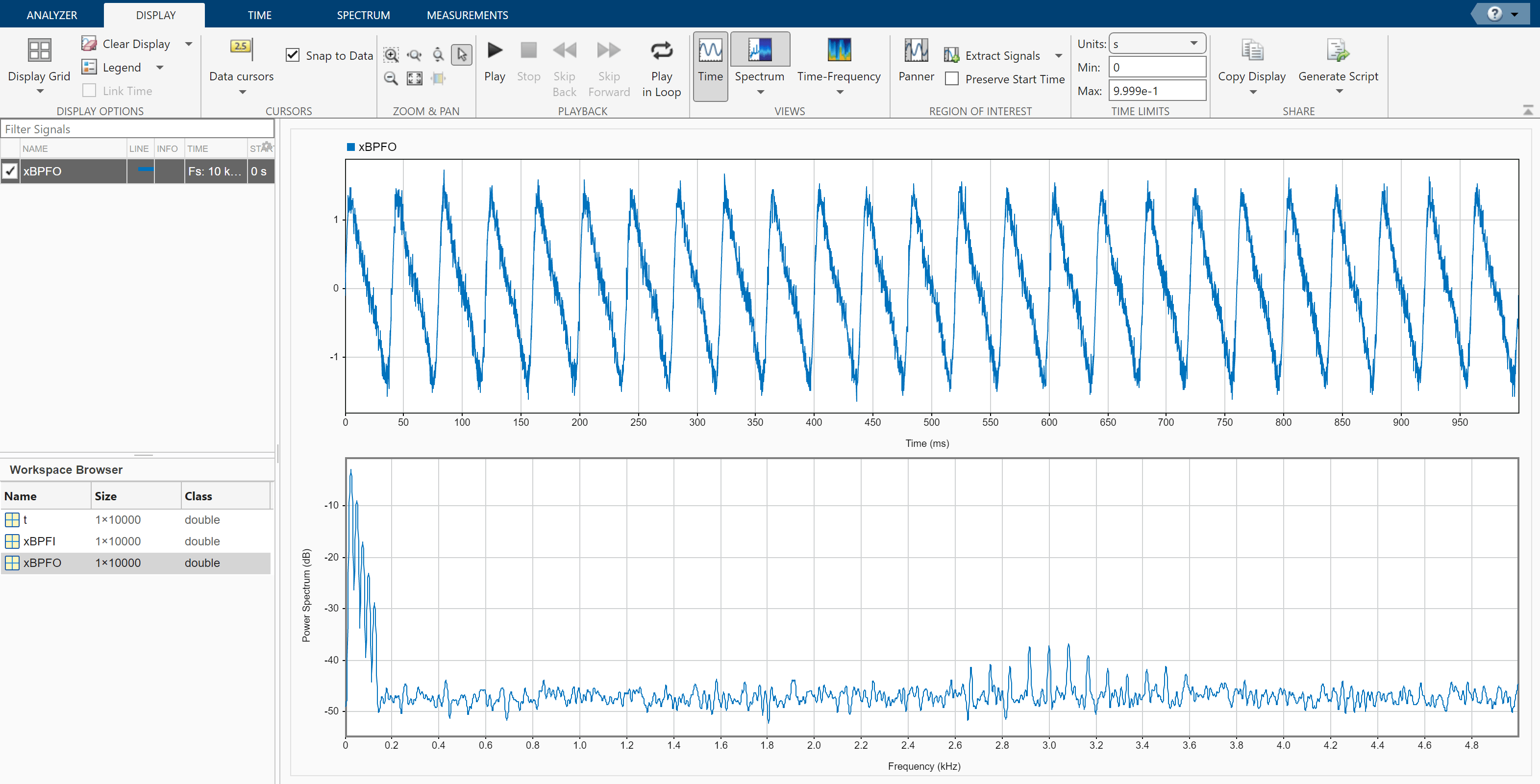Select the signal and, on the Analyzer tab, click Duplicate to generate a copy of it. Give the new signal the name `envspec` and drag it to the display. Compute the envelope spectrum of the signal using the Hilbert transform:

1. Remove the DC value of the signal. On the Analyzer tab, click Preprocessing ▼ and select Detrend. On the Detrend tab that appears, select `Constant` as the method. Click Detrend.

2. Bandpass-filter the detrended signal. On the Analyzer tab, click Preprocessing ▼ and select Bandpass. On the Bandpass tab that appears, enter 2250 Hz and 3750 Hz as the lower and upper passband frequencies, respectively. Click Bandpass.

3. Compute the envelope of the filtered signal. On the Analyzer tab, click Preprocessing ▼ and select Envelope. On the Envelope tab that appears, select `Hilbert` as the method. Click Envelope.

4. Remove the DC value of the envelope using Detrend.

The envelope spectrum appears in the spectrum view of the display. The envelope spectrum clearly displays the BPFO harmonics.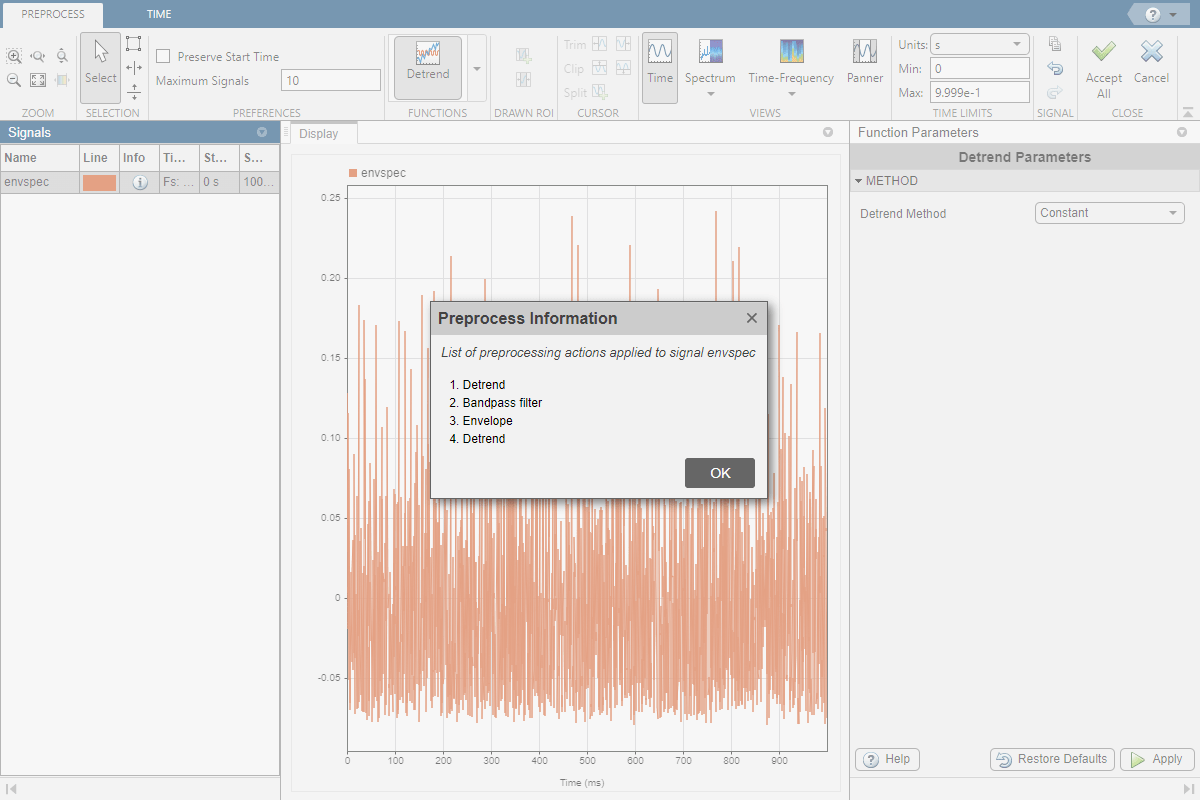Steps to Create an Integrated Analysis Script

The computation of the envelope spectrum can get tedious if it has to be repeated for many different bearings. Signal Analyzer can generate MATLAB® scripts and functions to help you automate the computation.

As an exercise, repeat the previous analysis for the BPFI signal. Signal Analyzer generates two components useful for the automation:

1. A function that preprocesses the signal by detrending it, filtering it, and computing its envelope

2. A script that computes the envelope spectrum

To create the integrated analysis script, put the preprocessing function and the plotting script together unchanged in a single file. (Alternatively, you can save functions in separate files.)

• If you save the script and the function in a single MATLAB® script, keep in mind that functions must appear at the end.

• You must add the keyword `end` at the end of each function.

1. Create Preprocessing Function

Initially, create the function that reproduces the preprocessing steps. Select the `envspec` signal. On the Analyzer tab, click Generate Function. The function, called `preprocess` by default, appears in the Editor. Save the generated function at the end of your integrated analysis script. The function expects a second argument specifying the time information. Preprocess the BPFI signal using the function.

`envspec = preprocess(xBPFI,t);`

2. Create Spectrum Script

In the app, remove the unprocessed signal from the display by clearing the check box next to its name. On the Display tab, click Generate Script ▼ and select `Spectrum Script`. The script appears in the Editor. Include the generated code in your integrated analysis script. When you run the analysis script, the generated spectrum script computes the envelope spectrum of the preprocessed BPFI signal.

```% Compute power spectrum % Generated by MATLAB(R) 9.6 and Signal Processing Toolbox 8.2. % Generated on: 12-Nov-2018 15:13:34 % Parameters timeLimits = [0 0.9999]; % seconds frequencyLimits = [0 5000]; % Hz %% % Index into signal time region of interest envspec_ROI = envspec(:); sampleRate = 10000; % Hz startTime = 0; % seconds minIdx = ceil(max((timeLimits(1)-startTime)*sampleRate,0))+1; maxIdx = floor(min((timeLimits(2)-startTime)*sampleRate,length(envspec_ROI)-1))+1; envspec_ROI = envspec_ROI(minIdx:maxIdx); % Compute spectral estimate % Run the function call below without output arguments to plot the results [Penvspec_ROI, Fenvspec_ROI] = pspectrum(envspec_ROI,sampleRate, ... 'FrequencyLimits',frequencyLimits);```

3. Plot Envelope Spectrum

Plot the envelope spectrum. Compare the peak locations to the frequencies of the first ten BPFI harmonics. You can also plot the envelope spectrum using the `pspectrum` command with no output arguments.

```plot(Fenvspec_ROI,(Penvspec_ROI)) hold on [X,Y] = meshgrid((1:10)*bpfi,ylim); plot(X,Y,':k') hold off xlim([0 10*bpfi])```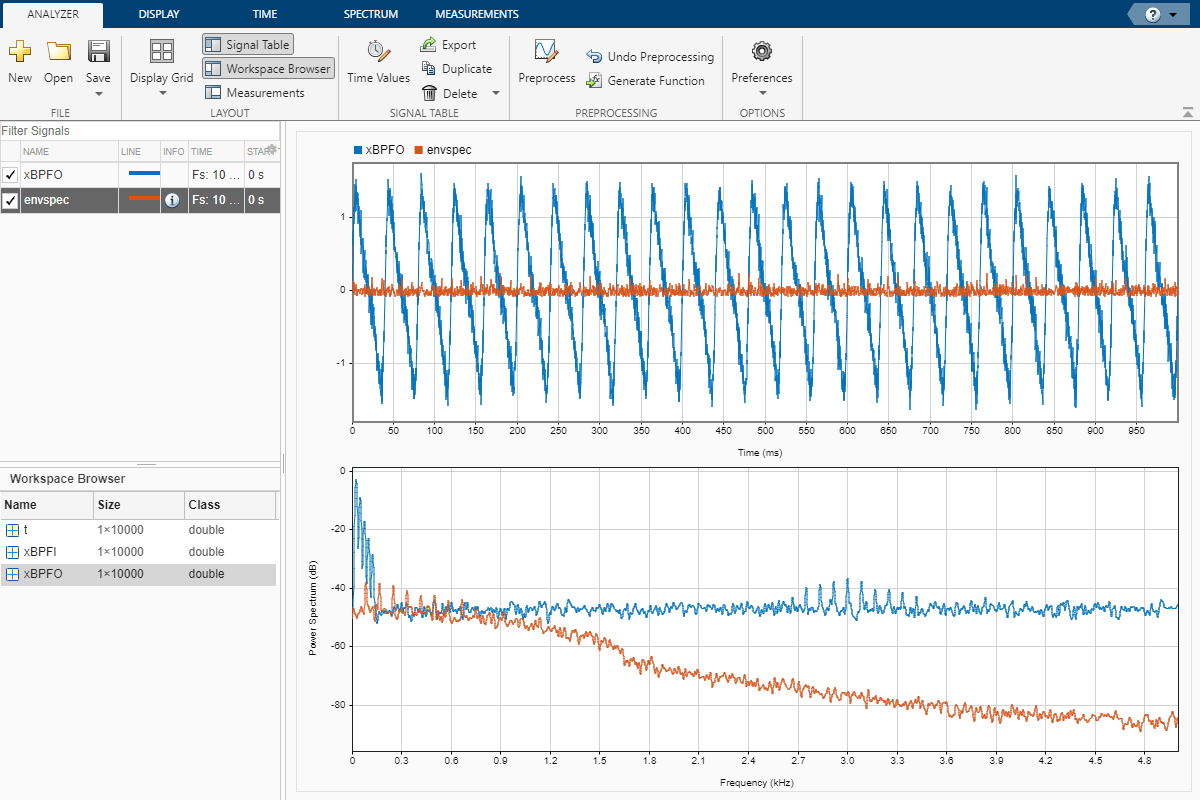Function Code

Signal Preprocessing Function

The signal preprocessing function generated by the app combines detrending, bandpass filtering, and envelope computation.

```function y = preprocess(x,tx) % Preprocess input x % This function expects an input vector x and a vector of time values % tx. tx is a numeric vector in units of seconds. % Generated by MATLAB(R) 9.6 and Signal Processing Toolbox 8.2. % Generated on: 12-Nov-2018 15:09:44 y = detrend(x,'constant'); Fs = 1/mean(diff(tx)); % Average sample rate y = bandpass(y,[2250 3750],Fs,'Steepness',0.85,'StopbandAttenuation',60); [y,~] = envelope(y); y = detrend(y,'constant'); end```

Bearing Data Generating Function

The bearing has pitch diameter $\mathit{p}=12$ cm and a bearing contact angle $\theta =0$. Each of the $\mathit{n}=8$ rolling elements has a diameter $\mathit{d}=2$ cm. The outer race remains stationary as the inner race is driven at ${\mathit{f}}_{0}=25$ cycles per second. An accelerometer samples the bearing vibrations at 10 kHz.

```function [t,xBPFO,xBPFI,bpfi] = bearingdata p = 0.12; d = 0.02; n = 8; th = 0; f0 = 25; fs = 10000;```

For a healthy bearing, the vibration signal is a superposition of several orders of the driving frequency, embedded in white Gaussian noise.

```t = 0:1/fs:1-1/fs; z = [1 0.5 0.2 0.1 0.05]*sin(2*pi*f0*[1 2 3 4 5]'.*t); xHealthy = z + randn(size(z))/10;```

A defect in the outer race causes a series of 5 millisecond impacts that over time result in bearing wear. The impacts occur at the ball pass frequency outer race (BPFO) of the bearing,

$\mathrm{BPFO}=\frac{1}{2}{\mathit{nf}}_{0}\left[1-\frac{\mathit{d}}{\mathit{p}}\mathrm{cos}\text{\hspace{0.17em}}\theta \right]$.

Model the impacts as a periodic train of 3 kHz exponentially damped sinusoids. Add the impacts to the healthy signal to generate the BPFO vibration signal.

```bpfo = n*f0/2*(1-d/p*cos(th)); tmp = 0:1/fs:5e-3-1/fs; xmp = sin(2*pi*3000*tmp).*exp(-1000*tmp); xBPFO = xHealthy + pulstran(t,0:1/bpfo:1,xmp,fs)/4;```

If the defect is instead in the inner race, the impacts occur at a frequency

$\mathrm{BPFI}=\frac{1}{2}{\mathit{nf}}_{0}\left[1+\frac{\mathit{d}}{\mathit{p}}\mathrm{cos}\text{\hspace{0.17em}}\theta \right]$.

Generate the BPFI vibration signal by adding the impacts to the healthy signals.

```bpfi = n*f0/2*(1+d/p*cos(th)); xBPFI = xHealthy + pulstran(t,0:1/bpfi:1,xmp,fs)/4; end```

Programmatic Use

expand all

`signalAnalyzer` opens the Signal Analyzer app.

`signalAnalyzer(sig)` opens the Signal Analyzer app and imports and plots the signal `sig`. If the app is already open, then it plots `sig` in the current display. If `sig` is already plotted but has changed, then the function call updates the plot.

`sig` can be a variable in the workspace or a MATLAB expression. `sig` can be:

See Data Types Supported by Signal Analyzer for more details.

By default, the app plots the signal as a function of sample index. If you provide time information, or if the signal has inherent time information, then the app plots the signal as a function of time.

`signalAnalyzer(sig1,...,sigN)` imports N signal vectors or matrices and plots them in the current display. The app does not support importing signals with inherent time information and signals without inherent time information in the same function call.

`signalAnalyzer(___,'SampleRate',fs)` specifies a sample rate, `fs`, as a positive scalar expressed in Hz. The app uses the sample rate to plot one or more signals against time, assuming a start time of zero. You can specify a sample rate for signals with no inherent time information.

`signalAnalyzer(___,'SampleTime',ts)` specifies a sample time, `ts`, as a positive scalar expressed in seconds. The app uses the sample time to plot one or more signals against time, assuming a start time of zero. You can specify a sample time for signals with no inherent time information.

`signalAnalyzer(___,'StartTime',st)` specifies a signal start time, `st`, as a scalar expressed in seconds. If you do not specify a sample rate or sample time, then the app assumes a sample rate of 1 Hz. You can specify a start time for signals with no inherent time information.

`signalAnalyzer(___,'TimeValues',tv)` specifies a vector, `tv`, with time values corresponding to the data points. `tv` can be a real numeric vector with values expressed in seconds. `tv` can also be a `duration` array. The values in `tv` must be unique and cannot be `NaN`, but they need not be uniformly spaced. All input signals must have the same length as `tv`. You can specify a vector of time values for signals with no inherent time information.

Filtering and scalogram view do not support nonuniformly sampled signals.